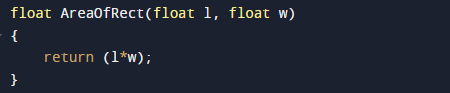# C++ Program to Calculate area of rectangle

In this tutorial you will learn about the C++ Program to Calculate area of rectangle and its application with practical example.

In this tutorial, we will learn to create a c++ program that find Area of Rectangle using c++ programming

## Prerequisites

Before starting with this tutorial we assume that you are best aware of the following C programming topics:

• C++ Operators.
• Functions.
• Basic input/output.
• Basic c++ programming.

## What Is Area?

Area measures the space inside any shape.
The extent or measurement of a surface or piece of land.
Area term is  used to define the amount of space cover up by a 2D shape or surface.

## C++ Program to Calculate area of rectangle

In this program , we will calculate Area of Rectangle of a given Length and Width .First of all user will be prompted to enter Length and Width and afterword passing these value to function to calculate Area of Rectangle.

## OutputIn the above program, we have first declared and initialized a set variables required in the program.

• length = it will hold entered length.
• width = it will hold entered width.
• l=  hold value of length.
• w=hold value of width.

Now let us look process of find area of rectangle step by step.

• first or all take length and width from user.
• then pass these value  of length and width to function AreaOfRect(length, width).
• where in function  we calculate area of rectangle using formula (a=l*w).• after, calculating area function returns us the area of rectangle or given length and width,
• Finally in main function we will print the area of rectangle of given values.

In this tutorial we have learn about the C++ Program to Calculate area of rectangle and its application with practical example. I hope you will like this tutorial.